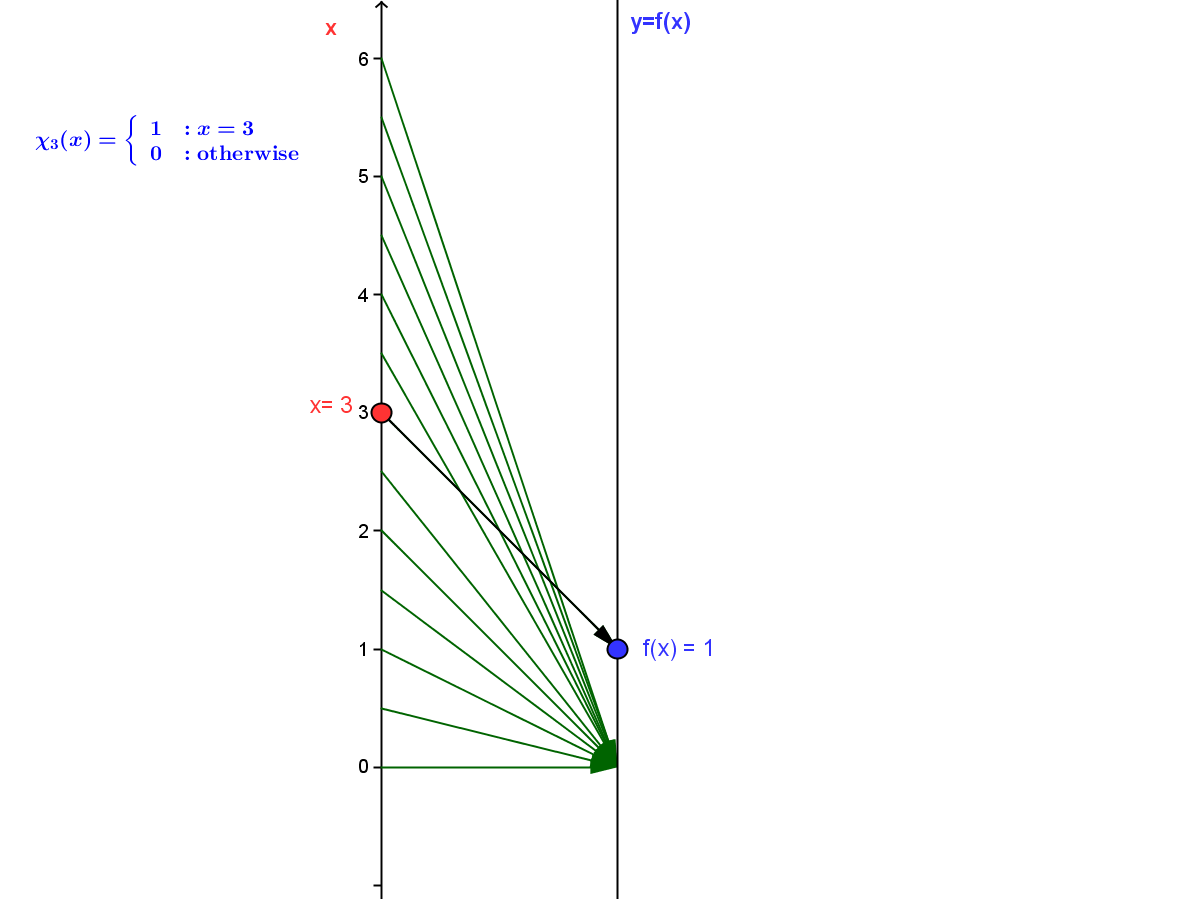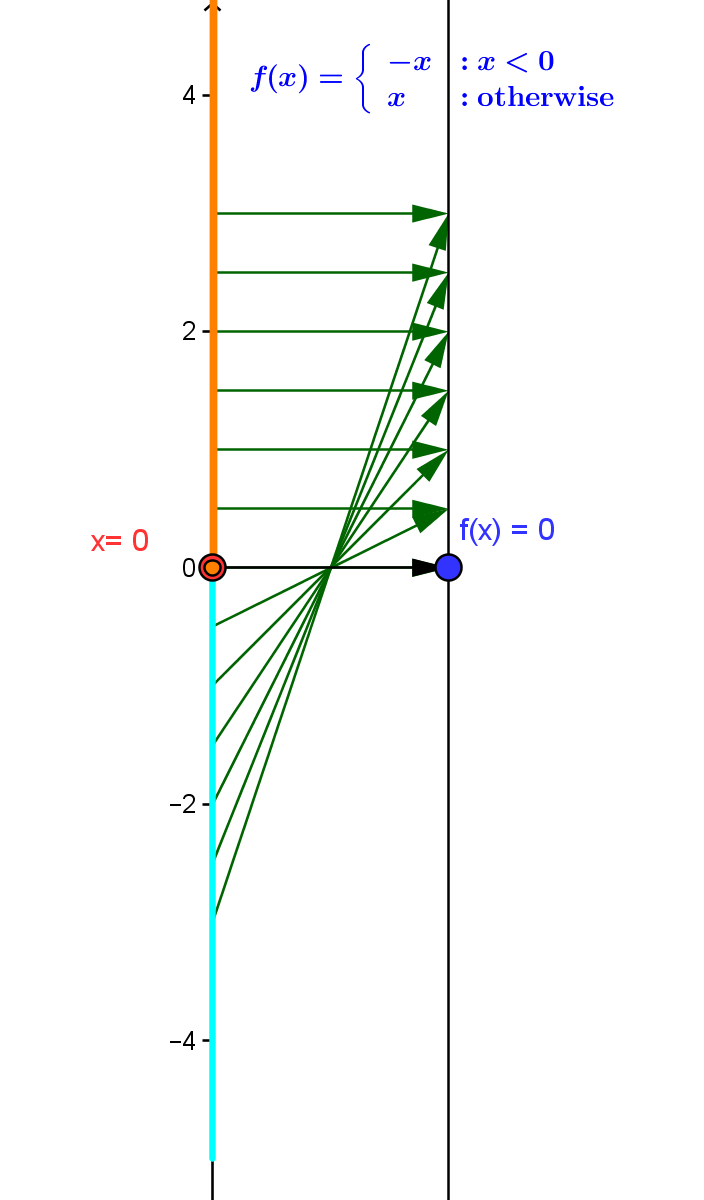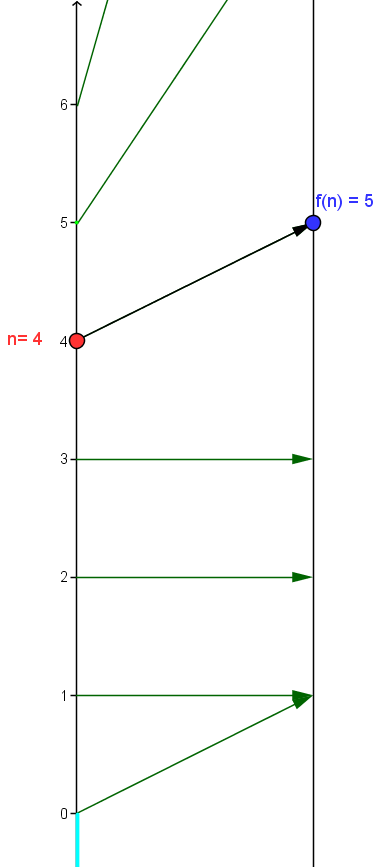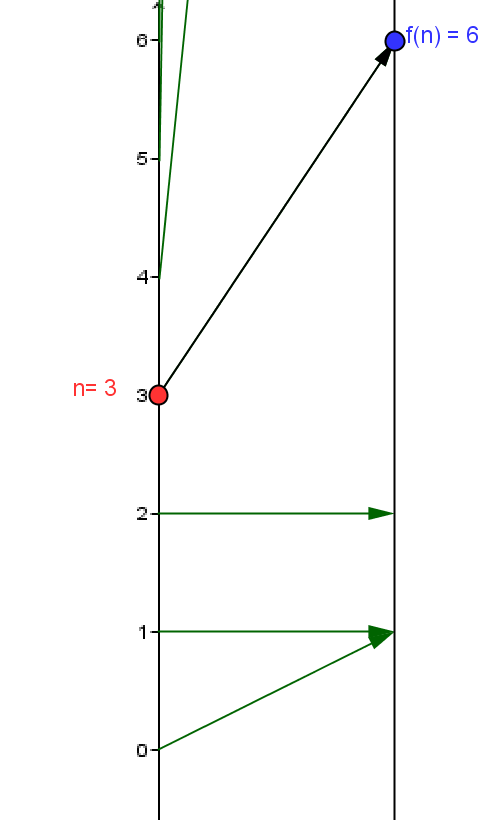### SectionOWOther Algebraic Functions

Other Ways to Define Functions

Introduction: Unlike polynomial functions and rational functions many functions are defined by techniques that rely on understanding more than the simple features of performing arithmetic on a single number.

Four common methods beyond simple arithmetic for defining functions are
1. Definition by piecewise cases;
2. Definition by function inversion;
3. Definition by use of equations with multiple solutions (implicit definition).
4. Definition by recursion or induction.

#### OW.FDPC Function Definition by Piecewise Cases

Functions defined by piecewise cases are characterized by partitioning the domain of the function and defining the function value by identifying in what particular subset of the partition a number lies. Wikipedia on piecewise-defined functions.

A very simple example of a function defined by piecewise cases is the function $\chi_3$ where $\chi_3(3) = 1$ and $\chi_3(x) = 0$ for any $x \ne 3$.
[This function $\chi _3$  is described as "the characteristic function of the number $3$."]Mapping Diagram for $\chi_3$

Another important example of a function defined by piecewise cases is the function $f(x)= |x|$ where $f(x) = x$ when $x \ge 0$ and $f(x)=-x$ when $x < 0$.Mapping Diagram for $f(x) = |x|$
Functions defined by piecewise cases are abundant and important in many applications.

OW.FDINV Function Definition by Inversion

We have already discussed inverse functions and mapping diagrams in the section LF.INV on inverses of linear functions. See also Wikipedia on Inverse Functions.

We investigate definition by inversion further by exploring the core positive power functions $P_n(x) = x^n$ and their "inverses. "

Comment: Consider the core power function $P_3$ where $P_3(x) =x^3$.  This  function has a unique inverse function which we denote by $InvP_3$ or $P_3 ^{-1}$. It is commonly know as the "cube root" function so $InvP_3(x)= P_3 ^{-1}(x) = \sqrt x$ . The property that defines $invP_3=P_3 ^{-1}$ is  that $P_3(P_3 ^{-1}(x)) = (\sqrt x)^3 = x$ for all numbers $x$. We can also understand this by saying $P_3 ^{-1}(s)$ is defined as the unique number $t$ where $P_3(t) = t^3 =s$.

Applications of this method are studied extensively in intermediate algebra (for logarithms) and trigonometry ( for inverse trig functions).

#### OW.FDImpl  Implicit Function Definition

Functions implicitly defined by an equation are not mentioned in beginning algebra courses but occur quietly in the background connections between linear (line) and quadratic (conic curve) equations and functions. Wikipedia on Implicit Functions.

1. A very revealing example is given by recognizing an inverse function for the function $f$ is an implicit function for the equation $f(y)-x = 0$.
2. A commonly encountered example is given by recognizing that  the function $f(x) = \sqrt{ 1- x^2}$ for $x \in [-1,1]$  is an implicit function for the equation $x^2 +y^2 - 1 = 0$.

Example OW.0  An Implicit (Piecewise) Case Defined Function.

This example presents a (piecewise) case defined function $f$, implicitly defined with respect to the equation $x^2 +y^2 -4 = 0$ with a table of data,  a graph and a mapping diagram.

#### OW.FDRec  Recursive Function Definition

Functions defined recursively  (inductively) on subsets of the integers or related subsets of the real numbers are usually not mentioned directly in algebra courses, yet are encountered through experience in these and later courses both with sequences of numbers and of functions. Wikipedia on Recursive Definition of Functions

Examples of recursive functions $f: N \rightarrow R$ include the factorial function defined by $f(0)= 1$ and $f(n+1) = (n+1) \times f(n)$ for all $n > 0$ and  the Fibonacci sequence function, defined by $f(0)=0$, $f(1)= 1$ and $f(n+2) = f(n) +f(n+1)$. Both these functions can be visualized by mapping diagrams.

Fibonacci Function
 $n$ $f(n+2) = f(n) +f(n+1)$ 0 1 1 1 2 2 3 3 4 5 5 8Factorial Function
 $n$ $f(n+1)=(n+1)*f(n)$ 0 1 1 1 2 2 3 6 4 24 5 120Treatment of functions defined by cases and by inverses with their graphical interpretation is common in algebra courses in preparation for calculus.
Functions defined implicitly by equations or recursively are not mentioned much, if at all, before a first course in calculus.

What is missing is a balanced treatment using mapping diagrams to reinforce the function aspect in the visualizations of these other ways to define functions.  That will be emphasis of this section. It will provide examples that will help students use the power of the mapping diagram along with the three other tools (equations, tables , and graphs) to understand these other ways to define functions.